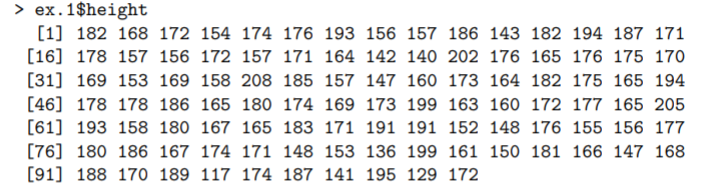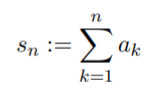# Numeric Sequence

A numeric sequence is a list of ordered numbers. A couple of simple examples: 1, 2, 3 or 99, 89, 79.

The geometric sequence is a well-known numeric sequence in calculus. Each term in the sequence is found by multiplying (or dividing) by the same value. For example, the sequence 1, 2, 4, 8, 16 is geometric because each number is a result of multiplying the one before it by 2 (e.g. 1 * 2 = 2, 2 * 2 = 4).

The numbers don’t have to be in ascending or descending order to be called a “numeric sequence”. They just have to be in some order than makes sense for the purpose at hand. For example, the following numeric sequence was used to create a histogram :## Formal Definition of a Numeric Sequence

In calculus, a numeric sequence is usually defined a little more formally. First, the numbers in the sequence must be real numbers.
Second, each term in the sequence must be a result of either adding or subtracting the term before it. Formally, that is stated as:

• s1: = a1
• s2: = a1 + a2
• s3: = a1 + a2 + a3Or, it can be more simply represented by the following summation notation:## Numeric Sequence in Computational Logic

Computational logic uses computers to establish facts in a logical formalism . The facts are defined much more precisely than simple “a sequence of ordered numbers”:

A sequence of characters is a numeric sequence if and only if one of the following is true :

1. The sequence is an optionally signed (±) base 10 digit sequence which may or may not have a dot character on the right-hand side.
2. The sequence is a #-sequence. A #-sequence has a length of three or more. The first character is always #. The numeric value is the sequence’s base n signed value found by deleting the first two characters. “n” 2, 8, or 16 depending on whether the second character of the sequence is is B, O, or X).

## References

 Yakir, B. (2011). Introduction to Statistical Thinking (With R, Without Calculus). Retrieved April 3, 2021 from: https://my.uopeople.edu/pluginfile.php/57436/mod_book/chapter/37629/MATH1280.IntroStat.pdf
 Proceedings of the Royal Society. Computational logic: its origins and applications. https://royalsocietypublishing.org/doi/10.1098/rspa.2017.0872
 Boyer, R. & Moore, S. A Precise Description of a Computational Logic. Retrieved April 3, 2021 from: https://www.cs.utexas.edu/users/boyer/ftp/cs.389r.course.packet.pdf

CITE THIS AS:
Stephanie Glen. "Numeric Sequence" From StatisticsHowTo.com: Elementary Statistics for the rest of us! https://www.statisticshowto.com/numeric-sequence/